Products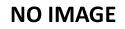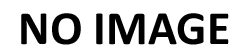### A10132

(image does not represent actual size)
Dimensions: 3/4" (W) x 1" (H)
A10132### A10133

(image does not represent actual size)
Dimensions: 7/8" (W) x 7/8" (H)
A10133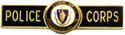### A10269

(image does not represent actual size)
Dimensions: 5/16" (W) x 1 3/4" (H)
A10269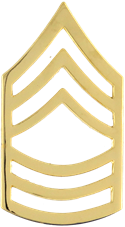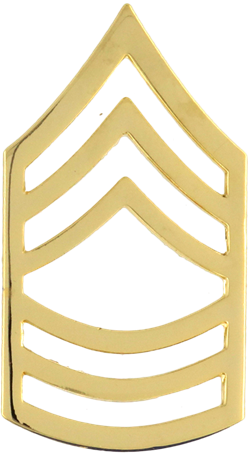### A11315

(image does not represent actual size)
Dimensions: 3/4" (W) x 1 1/2" (H)
A11315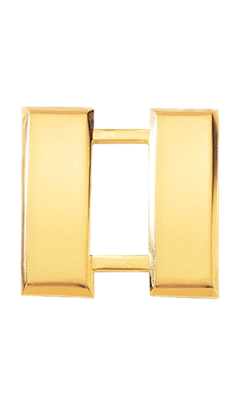### A1140

(image does not represent actual size)
Dimensions: 1" (W) x 1" (H)
A1140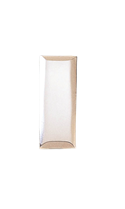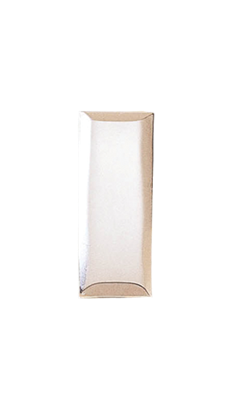### A1141

(image does not represent actual size)
Dimensions: 3/8" (W) x 1" (H)
A1141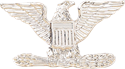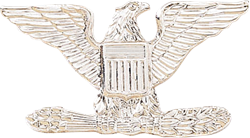### A1142

(image does not represent actual size)
Dimensions: 3/4" (W) x 1 1/2" (H)
A1142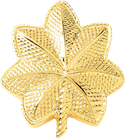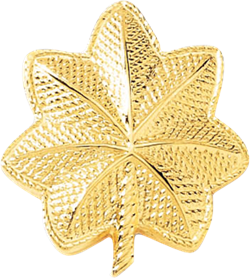### A1143

(image does not represent actual size)
Dimensions: 1 1/4" (W) x 1" (H)
A1143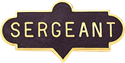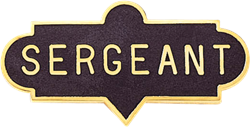### A1176

(image does not represent actual size)
Dimensions: 3/4" (W) x 1 1/2" (H)
A1176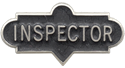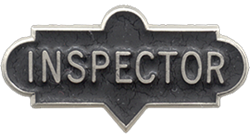### A1177

(image does not represent actual size)
Dimensions: 3/4" (W) x 1 1/2" (H)
A1177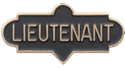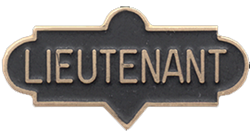### A1178

(image does not represent actual size)
Dimensions: 3/4" (W) x 1 1/2" (H)
A1178### A1180

(image does not represent actual size)
Dimensions: 1/4" (W) x 3/4" (H)
A1180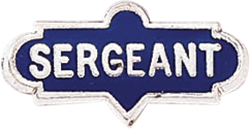### A1190

(image does not represent actual size)
Dimensions: 1/4" (W) x 3/4" (H)
A1190### A1191

(image does not represent actual size)
Dimensions: 1/4" (W) x 3/4" (H)
A1191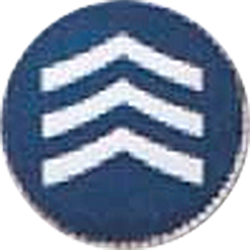### A12445

(image does not represent actual size)
Dimensions: 11/16" (D)
A12445